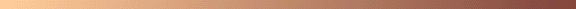PSEUDOPALINDROMES```Let b be a numeric base (such as 10).  Let S be a set of positive
integers such that

I.  2(number of elements of S)+1 < b (for base b = 10, this
implies that S has at most 4 elements).

II. Every member of S is either the sum or difference of two
distinct members of S (which implies that the S must contain
at least 3 elements).

Then

N(b, S) = b^max(S) ( (SUM, s in S, b^s) - 1 + (SUM, s in S, b^-s) )

is the pseudopalindrome base of a palindromic square.

Condition I guarantees that squaring N(b, S) will produce no carries.
Condition II guarantees that when squaring N(b, S), all columns will
be positive.

For example, let b = 10.  Then set S = { 3, 5, 8 } meets conditions
I and II above.  We compute

N(10, S)
= 10^8 * (10^8 + 10^5 + 10^3 - 1 + 10^-3 + 10^-5 + 10^-8)
= 10010100n00101001
= 10010099900101001

which is the pseudopalindrome base of the palindromic square

100202100010002070200010001202001

(in the pseudopalindromic representation 10010100n00101001, note
that the 1's occur at the 3rd, 5th, and 8th positions to left and
right of the central "n" digit of value -1).

Similarly, the set S = { 1, 2, 4, 5 } meets conditions I and II above,
so that

11010911011^2 = 121240161292161042121

is a palindrome.

However, S = { 1, 2, 3, 4, 5 } does not meet condition I, since
2(5)+1 = 11 >= b = 10.  Thus

11111n11111^2
= 11110911111^2
= 123452345716543254321

is not palindromic (the central digit carries).

S = { 1, 2, 5, 6 } meets condition I, but not Condition II, since
2 is not the sum or difference of distinct members of S.  Hence

110011n110011
1100109110011^2 = 1210240053929194500420121

is not palindromic (some columns in the pseudopalindromic